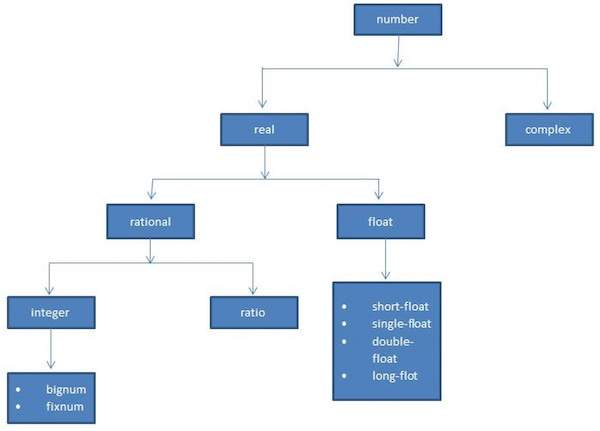# LISP - Numbers

Common Lisp defines several kinds of numbers. The number data type includes various kinds of numbers supported by LISP.

The number types supported by LISP are −

• Integers
• Ratios
• Floating-point numbers
• Complex numbers

The following diagram shows the number hierarchy and various numeric data types available in LISP −## Various Numeric Types in LISP

The following table describes various number type data available in LISP −

Sr.No. Data type & Description
1

fixnum

This data type represents integers which are not too large and mostly in the range -215 to 215-1 (it is machine-dependent)

2

bignum

These are very large numbers with size limited by the amount of memory allocated for LISP, they are not fixnum numbers.

3

ratio

Represents the ratio of two numbers in the numerator/denominator form. The / function always produce the result in ratios, when its arguments are integers.

4

float

It represents non-integer numbers. There are four float data types with increasing precision.

5

complex

It represents complex numbers, which are denoted by #c. The real and imaginary parts could be both either rational or floating point numbers.

### Example

Create a new source code file named main.lisp and type the following code in it.

```(write (/ 1 2))
(terpri)
(write ( + (/ 1 2) (/ 3 4)))
(terpri)
(write ( + #c( 1 2) #c( 3 -4)))
```

When you execute the code, it returns the following result −

```1/2
5/4
#C(4 -2)
```

## Number Functions

The following table describes some commonly used numeric functions −

Sr.No. Function & Description
1

+, -, *, /

Respective arithmetic operations

2

sin, cos, tan, acos, asin, atan

Respective trigonometric functions.

3

sinh, cosh, tanh, acosh, asinh, atanh

Respective hyperbolic functions.

4

exp

Exponentiation function. Calculates ex

5

expt

Exponentiation function, takes base and power both.

6

sqrt

It calculates the square root of a number.

7

log

Logarithmic function. It one parameter is given, then it calculates its natural logarithm, otherwise the second parameter is used as base.

8

conjugate

It calculates the complex conjugate of a number. In case of a real number, it returns the number itself.

9

abs

It returns the absolute value (or magnitude) of a number.

10

gcd

It calculates the greatest common divisor of the given numbers.

11

lcm

It calculates the least common multiple of the given numbers.

12

isqrt

It gives the greatest integer less than or equal to the exact square root of a given natural number.

13

floor, ceiling, truncate, round

All these functions take two arguments as a number and returns the quotient; floor returns the largest integer that is not greater than ratio, ceiling chooses the smaller integer that is larger than ratio, truncate chooses the integer of the same sign as ratio with the largest absolute value that is less than absolute value of ratio, and round chooses an integer that is closest to ratio.

14

ffloor, fceiling, ftruncate, fround

Does the same as above, but returns the quotient as a floating point number.

15

mod, rem

Returns the remainder in a division operation.

16

float

Converts a real number to a floating point number.

17

rational, rationalize

Converts a real number to rational number.

18

numerator, denominator

Returns the respective parts of a rational number.

19

realpart, imagpart

Returns the real and imaginary part of a complex number.

### Example

Create a new source code file named main.lisp and type the following code in it.

```(write (/ 45 78))
(terpri)
(write (floor 45 78))
(terpri)
(write (/ 3456 75))
(terpri)
(write (floor 3456 75))
(terpri)
(write (ceiling 3456 75))
(terpri)
(write (truncate 3456 75))
(terpri)
(write (round 3456 75))
(terpri)
(write (ffloor 3456 75))
(terpri)
(write (fceiling 3456 75))
(terpri)
(write (ftruncate 3456 75))
(terpri)
(write (fround 3456 75))
(terpri)
(write (mod 3456 75))
(terpri)
(setq c (complex 6 7))
(write c)
(terpri)
(write (complex 5 -9))
(terpri)
(write (realpart c))
(terpri)
(write (imagpart c))
```

When you execute the code, it returns the following result −

```15/26
0
1152/25
46
47
46
46
46.0
47.0
46.0
46.0
6
#C(6 7)
#C(5 -9)
6
7
```